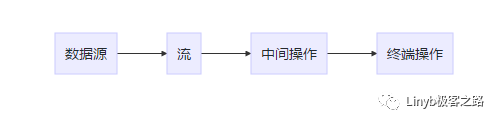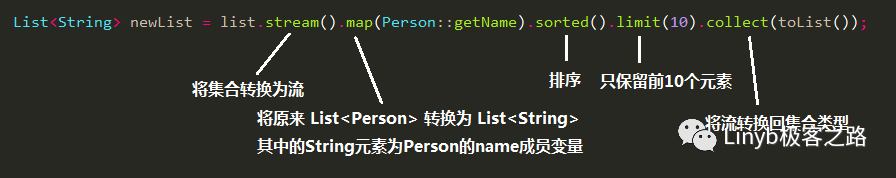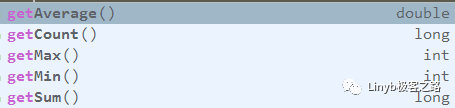• 如果您觉得本站非常有看点，那么赶紧使用Ctrl+D 收藏吧

# Java8 Stream + +很不错的文章集合

https://cloud.tencent.com/developer/article/1187833

Java 8 发布至今也已经好几年过去，如今 Java 也已经向 11 迈去，但是 Java 8 作出的改变可以说是革命性的，影响足够深远，学习 Java 8 应该是 Java 开发者的必修课。 今天给大家带来 Java 8 Stream 讲解，为什么直接讲这个，是因为只要你学完，立刻就能上手，并能让它在你的代码中大展身手。 值得注意的是：学习 Stream 之前必须先学习 lambda 的相关知识。本文也假设读者已经掌握 lambda 的相关知识。

### 本篇文章主要内容：

• 介绍 Stream 以及 Stream 是如何处理集合的
• 介绍 Stream 与集合的关系与区别

# 一. 什么是 Stream

Stream 中文称为 “流”，通过将集合转换为这么一种叫做 “流” 的元素序列，通过声明性方式，能够对集合中的每个元素进行一系列并行或串行的流水线操作。

# 二. 流操作# 三. 流与集合

### 什么时候计算

Stream 和集合的其中一个差异在于什么时候进行计算。

### 外部迭代和内部迭代

Stream 和集合的另一个差异在于迭代。

Java 8 引入 Stream 很大程度是因为，流的内部迭代可以自动选择一种合适你硬件的数据表示和并行实现；而以往程序员自己进行 foreach 之类的时候，则需要自己去管理并行等问题。

### 一次性的流

```Stream<String> stream = list.stream().map(Person::getName).sorted().limit(10);
List<String> newList = stream.collect(toList());
List<String> newList2 = stream.collect(toList());```

## 四. 关于并行

Stream 让程序员能够比较轻易地实现对数据集合的并行处理，但要注意的是，不是所有情况的适合，有些时候并行甚至比顺序进行效率更低，而有时候因为线程安全问题，还可能导致数据的处理错误，这些我会在下一篇文章中讲解。

### 本篇文章主要内容：

• 流基本的常用方法
• 一种特化形式的流——数值流
• Optional 类
• 如何构建一个流
• collect 方法
• 并行流相关问题

# 一. 一般方法

```List<Person> list = new ArrayList<>();

Person 类包含年龄和姓名两个成员变量

```private String name;
private int age;```

### 1. stream() / parallelStream()

```List list = new ArrayList();
// return Stream<E>
list.stream();```

### 2. filter(T -> boolean)

```保留年龄为 20 的 person 元素
list = list.stream()
.filter(person -> person.getAge() == 20)
.collect(toList());

collect(toList()) 可以把流转换为 List 类型，这个以后会讲解

### 4. sorted() / sorted((T, T) -> int)

```根据年龄大小来比较：
list = list.stream()
.sorted((p1, p2) -> p1.getAge() - p2.getAge())
.collect(toList());```

```list = list.stream()
.sorted(Comparator.comparingInt(Person::getAge))
.collect(toList());```

### 5. limit(long n)

```list = list.stream()
.limit(2)
.collect(toList());

### 6. skip(long n)

```list = list.stream()
.skip(2)
.collect(toList());

tips:

• 用在 limit(n) 前面时，先去除前 m 个元素再返回剩余元素的前 n 个元素
• limit(n) 用在 skip(m) 前面时，先返回前 n 个元素再在剩余的 n 个元素中去除 m 个元素
```list = list.stream()
.limit(2)
.skip(1)
.collect(toList());

### 7. map(T -> R)

`List<String> newlist = list.stream().map(Person::getName).collect(toList());`

newlist 里面的元素为 list 中每一个 Person 对象的 name 变量

### 8. flatMap(T -> Stream

```List<String> list = new ArrayList<>();

list = list.stream().map(s -> s.split(" ")).flatMap(Arrays::stream).collect(toList());```

### 9. anyMatch(T -> boolean)

```是否存在一个 person 对象的 age 等于 20：
boolean b = list.stream().anyMatch(person -> person.getAge() == 20);```

### 12. findAny() 和 findFirst()

• findAny()：找到其中一个元素 （使用 stream() 时找到的是第一个元素；使用 parallelStream() 并行时找到的是其中一个元素）
• findFirst()：找到第一个元素

### 13. reduce((T, T) -> T) 和 reduce(T, (T, T) -> T)

```计算年龄总和：
int sum = list.stream().map(Person::getAge).reduce(0, (a, b) -> a + b);

int sum = list.stream().map(Person::getAge).reduce(0, Integer::sum);```

```计算年龄总乘积：
int sum = list.stream().map(Person::getAge).reduce(1, (a, b) -> a * b);```

`Optional<Integer> sum = list.stream().map(Person::getAge).reduce(Integer::sum);`

### 15. forEach()

```### 16. unordered()

list.stream().forEach(System.out::println);```

```向数据库插入新元素：
list.stream().forEach(PersonMapper::insertPerson);```

# 二. 数值流

## 1. 流与数值流的转换

• mapToInt(T -> int) : return IntStream
• mapToDouble(T -> double) : return DoubleStream
• mapToLong(T -> long) : return LongStream
`IntStream intStream = list.stream().mapToInt(Person::getAge);`

`LongStream longStream = list.stream().mapToInt(Person::getAge);`

`Stream<Integer> stream = intStream.boxed();`

• sum()
• max()
• min()
• average() 等…

## 3. 数值范围

IntStream 与 LongStream 拥有 range 和 rangeClosed 方法用于数值范围处理

• IntStream ： rangeClosed(int, int) / range(int, int)
• LongStream ： rangeClosed(long, long) / range(long, long)

• rangeClosed(1, 100) ：[1, 100]
• range(1, 100) ：[1, 100)

```求 1 到 10 的数值总和：
IntStream intStream = IntStream.rangeClosed(1, 10);
int sum = intStream.sum();```

# 三. Optional 类

NullPointerException 可以说是每一个 Java 程序员都非常讨厌看到的一个词，针对这个问题， Java 8 引入了一个新的容器类 Optional，可以代表一个值存在或不存在，这样就不用返回容易出问题的 null。之前文章的代码中就经常出现这个类，也是针对这个问题进行的改进。

Optional 类比较常用的几个方法有：

• isPresent() ：值存在时返回 true，反之 flase
• get() ：返回当前值，若值不存在会抛出异常
• orElse(T) ：值存在时返回该值，否则返回 T 的值

Optional 类还有三个特化版本 OptionalInt，OptionalLong，OptionalDouble，刚刚讲到的数值流中的 max 方法返回的类型便是这个

Optional 类其中其实还有很多学问，讲解它说不定也要开一篇文章，这里先讲那么多，先知道基本怎么用就可以。

# 四. 构建流

## 1. 值创建流

• Stream.of(T…) ： Stream.of(“aa”, “bb”) 生成流
```生成一个字符串流
Stream<String> stream = Stream.of("aaa", "bbb", "ccc");```
• Stream.empty() : 生成空流

## 2. 数组创建流

• Arrays.stream(T[ ])
• Arrays.stream(int[ ])
• Arrays.stream(double[ ])
• Arrays.stream(long[ ])

```只取索引第 1 到第 2 位的：
int[] a = {1, 2, 3, 4};
Arrays.stream(a, 1, 3).forEach(System.out :: println);

## 3. 文件生成流

`Stream<String> stream = Files.lines(Paths.get("data.txt"));`

## 4. 函数生成流

• iterate ： 依次对每个新生成的值应用函数
• generate ：接受一个函数，生成一个新的值
```Stream.iterate(0, n -> n + 2)

Stream.generate(Math :: random)

Stream.generate(() -> 1)

# 五. collect 收集数据

coollect 方法作为终端操作，接受的是一个 Collector 接口参数，能对数据进行一些收集归总操作

## 1. 收集

• toList
• toSet
• toCollection
`List newlist = list.stream.collect(toList());`

## 2. 汇总

### （1）counting

`long l = list.stream().collect(counting());`

`long l = list.stream().count();`

### （2）summingInt ，summingLong ，summingDouble

summing，没错，也是计算总和，不过这里需要一个函数参数

`int sum = list.stream().collect(summingInt(Person::getAge));`

`int sum = list.stream().mapToInt(Person::getAge).sum();`

`int sum = list.stream().map(Person::getAge).reduce(Interger::sum).get();`

### （3）averagingInt，averagingLong，averagingDouble

`Double average = list.stream().collect(averagingInt(Person::getAge));`

`OptionalDouble average = list.stream().mapToInt(Person::getAge).average();`

### （4）summarizingInt，summarizingLong，summarizingDouble

`IntSummaryStatistics l = list.stream().collect(summarizingInt(Person::getAge));`

IntSummaryStatistics 包含了计算出来的平均值，总数，总和，最值，可以通过下面这些方法获得相应的数据## 3. 取最值

maxBy，minBy 两个方法，需要一个 Comparator 接口作为参数

`Optional<Person> optional = list.stream().collect(maxBy(comparing(Person::getAge)));`

`Optional<Person> optional = list.stream().max(comparing(Person::getAge));`

## 4. joining 连接字符串

```String s = list.stream().map(Person::getName).collect(joining());

```String s = list.stream().map(Person::getName).collect(joining(","));

joining 还有一个比较特别的重载方法：

```String s = list.stream().map(Person::getName).collect(joining(" and ", "Today ", " play games."));

## 5. groupingBy 分组

groupingBy 用于将数据分组，最终返回一个 Map 类型

`Map<Integer, List<Person>> map = list.stream().collect(groupingBy(Person::getAge));`

### 多级分组

groupingBy 可以接受一个第二参数实现多级分组：

`Map<Integer, Map<T, List<Person>>> map = list.stream().collect(groupingBy(Person::getAge, groupBy(...)));`

### 按组收集数据

`Map<Integer, Integer> map = list.stream().collect(groupingBy(Person::getAge, summingInt(Person::getAge)));`

`groupingBy(Person::getAge)`

`groupingBy(Person::getAge, toList())`

## 6. partitioningBy 分区

```根据年龄是否小于等于20来分区
Map<Boolean, List<Person>> map = list.stream()
.collect(partitioningBy(p -> p.getAge() <= 20));

{
false=[Person{name='mike', age=25}, Person{name='tom', age=30}],
true=[Person{name='jack', age=20}]
}```

# 六. 并行

`int i = Stream.iterate(1, a -> a + 1).limit(100).parallel().reduce(0, Integer::sum);`

ArrayList

IntStream.range

Stream.iterate

HashSet

TreeSet